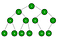# Visualization with pydot for beginners

How about drawing graphs and trees programmatically ?

Yes, that’s what we are going to learn in this post today. This post is for beginner’s who are very much interested in visualizations. So, today we’ll be attempting to draw the above recursion tree programmatically with pydot. But before that, we need to have some basic information:

i) pydot:

1. which is an interface to Graphviz,
2. can parse and dump into the DOT language used by GraphViz,
3. is written in pure Python, etc.

ii) Graph: It is a non-linear data structure consisting of nodes and edges. The nodes are sometimes also referred to as vertices and the edges are lines or arcs that connect any two nodes in the graph. More formally a Graph can be defined as,

“A Graph consists of a finite set of vertices(or nodes) and set of Edges which connect a pair of nodes”.

ii) Tree Data Structure: A tree is a nonlinear hierarchical data structure that consists of nodes connected by edges.Tree data structure

iii) Recursion Tree Method: Recursion Tree Method is a pictorial representation of an iteration method which is in the form of a tree where at each level nodes are expanded. In general, we consider the second term in recurrence as root. It is useful when the divide & Conquer algorithm is used.

Now, we’ll setup the environment for graphviz first. We can download the graphviz binary from here. Now we have to add graphviz bin folder to the environment variable path. If you are having trouble here is the installation guide.

Pip install pydot:

After installing pydot let’s try to draw some simple graphs, for that we can use any text editor of our choice, but I’ll be using VS Code.

Above code will create a simple graph like:

Looks cool!!!. Now, let’s say we want to show direction from node1 to node2 and from node1 to node3. We just need to change a single line and it should work fine.

To

And the output looks like:

Now, we want to have multiple edges between the same node. It can be easily done with the help of following single line of code.

The output looks like:

How to prevent another edge from drawing if it is already drawn ?

Here is the output:

Till now we got some basic idea on drawing edges, it’s time to explore how we can add some nodes on graph without edges.

Here is the output of above code:

# Now, is it possible to have create two nodes with same name ?

Here is the output of above code:

We’ve seen that we’re only able to get a single node with name “1”.So, our nodes always need a unique names, otherwise we cannot name them uniquely to attach edges between them. However, we can give each node a label, which is what is displayed when rendered. So, what exactly is a label? It is the value that appears on Node whereas the name is the actual value that identifies the node.

Here is the output of above code:

Adding different colors on nodes and edges to make our graphs more attractive:

Here is the output of above code:

Now it’s time to wrap up the tutorial by drawing some really cool graphs. We’ll try to draw some graphs found on google image results:

https://s3-ap-south-1.amazonaws.com/av-blog-media/wp-content/uploads/2018/09/Screenshot-from-2018-09-05-16-36-16.png

Here is the output of the above code:

The output looks like:

At last, we’ll try to visualize the recursion tree for nth Fibonacci number.

Updating the above code:

And the output looks like:

Looks cool !!!

We’ll be adding more nodes in upcoming posts. Keep following….for more updates.